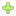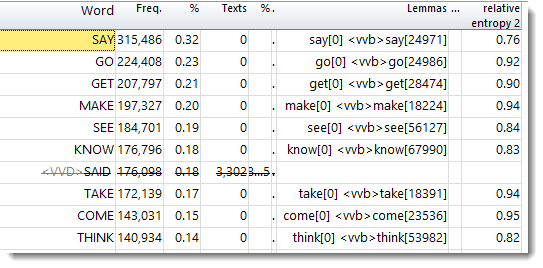# WordSmith Tools Manual

 Navigation: WordList > statistics relative entropy

The point of it...

The idea here is similar to dispersion, and it is measured over a series of frequencies such as those in a lemma.

The example given by Gries (2010) is the lemmas give and sing in English. It is clear from the table that some forms of sing (sings, sang, and sung) are infrequent, but no forms of give are very infrequent. The relative entropy value (0.91) for give tells you it is more smoothly spread over the 5 verb forms than sing is at 0.62.

 Rel. Entropy give 441 gives 105 giving 132 gave 175 given 376 0.91 sing 38 sings 2 singing 45 sang 3 sung 2 0.62

Using this function you can therefore compare lemmas in terms of how generally used or specialised all their lemma variants are.

How to compute it

This function suits lemmatised data, so it is not routinely shown for any word list. To compute relative entropy, you will need first to lemmatise at least some word forms in your word list.

Then choose the menu option Compute | Relative entropy.

a standard basis for the calculation

Choose a basis for the calculation. Here we choose 6 because there are exactly 6 verb types in the BNC data analysed (not 5 as in Gries's example).How to choose: comparing like with like

A new column will get created and compute a relative entropy value for each entry.

Results

Here is a BNC word list showing only verbs and computed relative entropy. MAKE scores higher (0.94) than SAY (0.76).High and low Relative Entropy scores